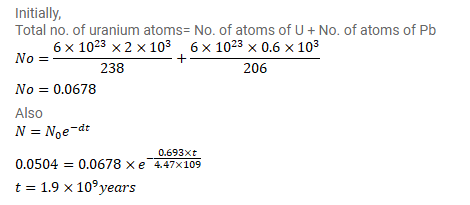# 238U decays to 206Pb with a half-life of 4.47×109y.

Question:

${ }^{238} \mathrm{U}$ decays to ${ }^{206} \mathrm{~Pb}$ with a half-life of $4.47 \times 10^{9} \mathrm{y}$. This happens in a number of steps. Can you justify a single half-life for this chain of processes? A sample of rock is found to contain $2.00 \mathrm{mg}$ of ${ }^{238} \mathrm{U}$ and $0.600 \mathrm{mg}$ of ${ }^{206} \mathrm{~Pb}$. Assuming that all the lead has come from uranium, find the life of the rock.

Solution: